Examples

Chapter 10 Class 11 Conic Sections
Serial order wise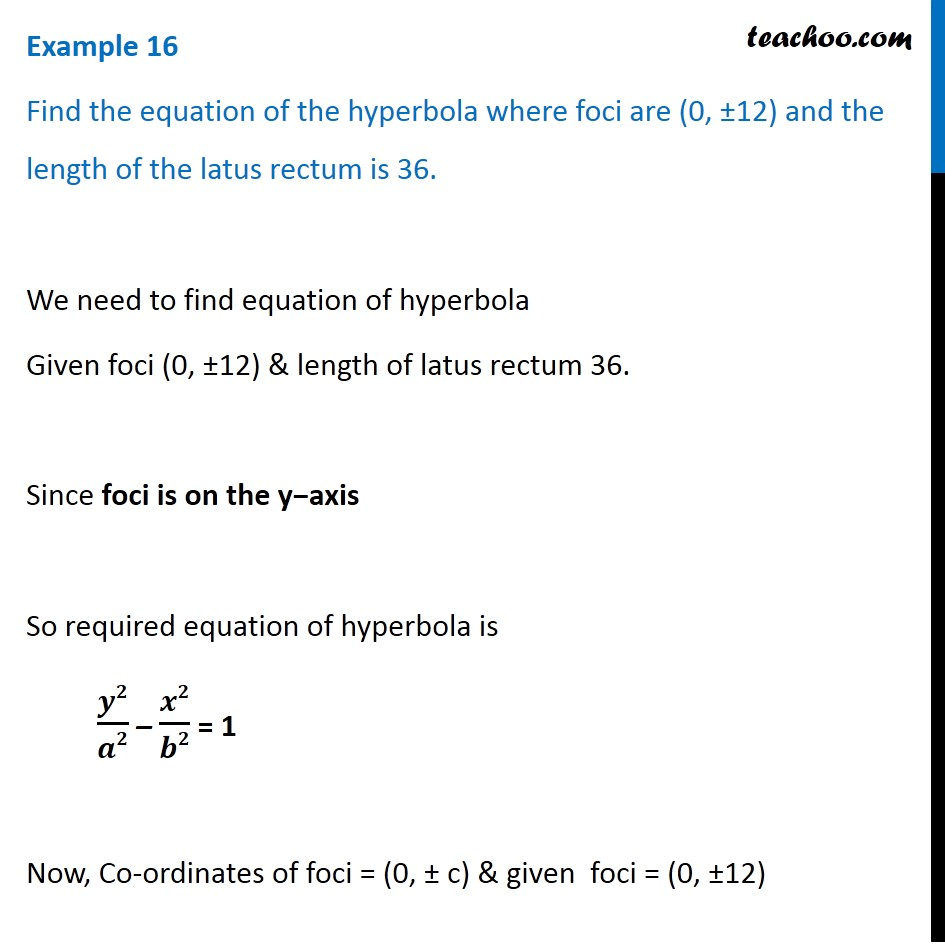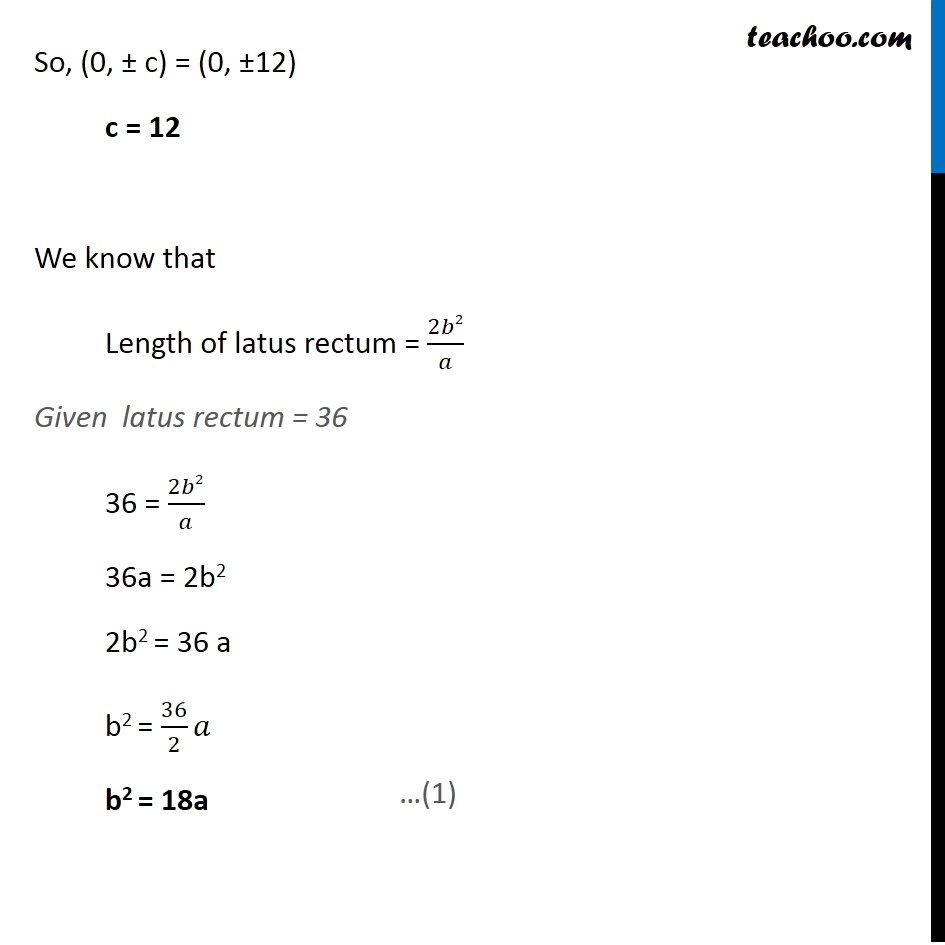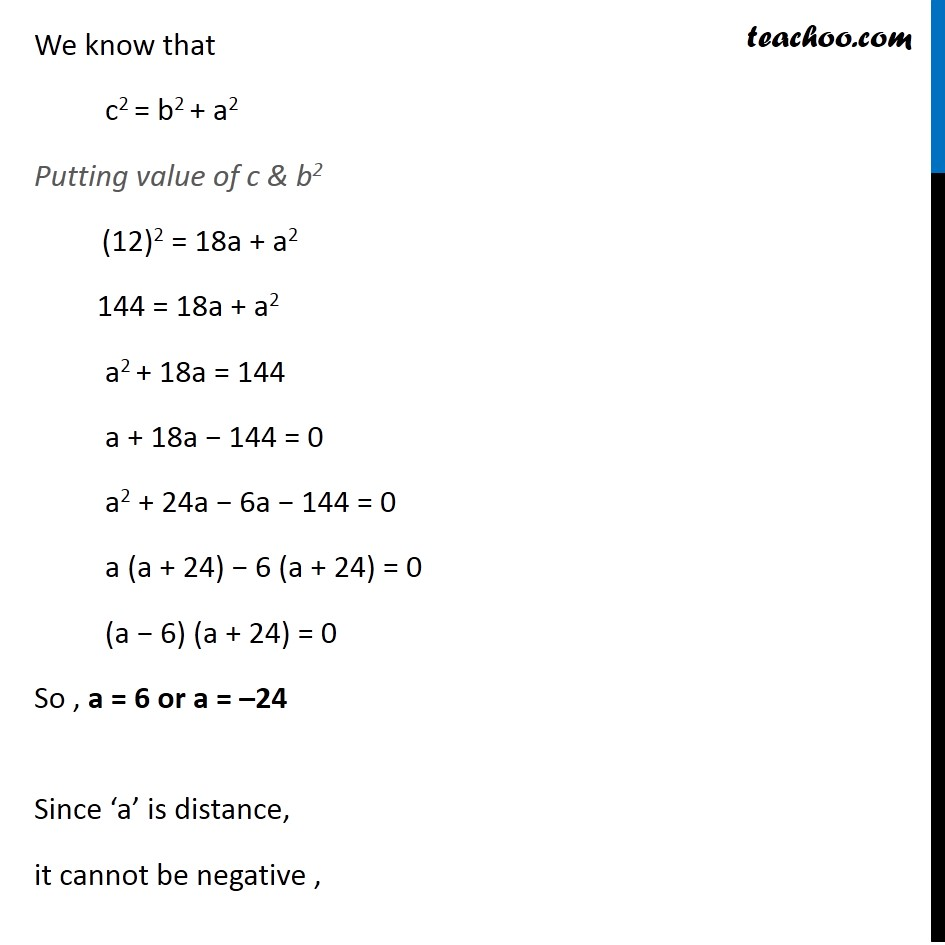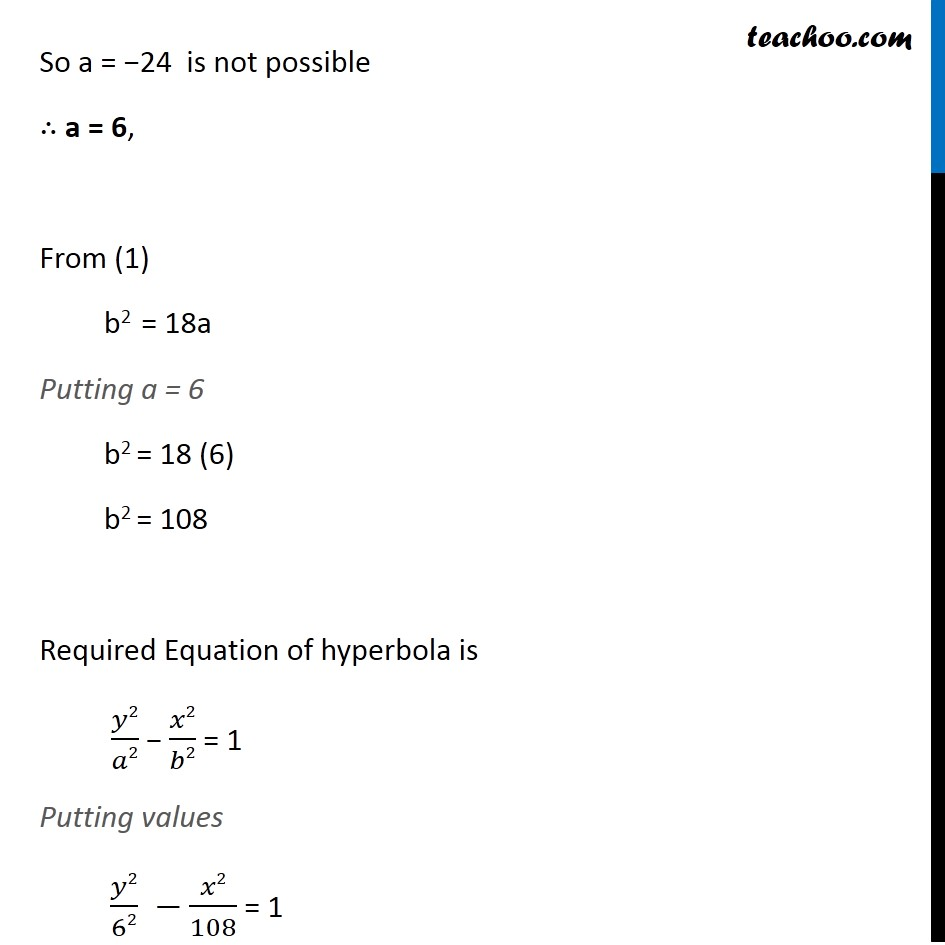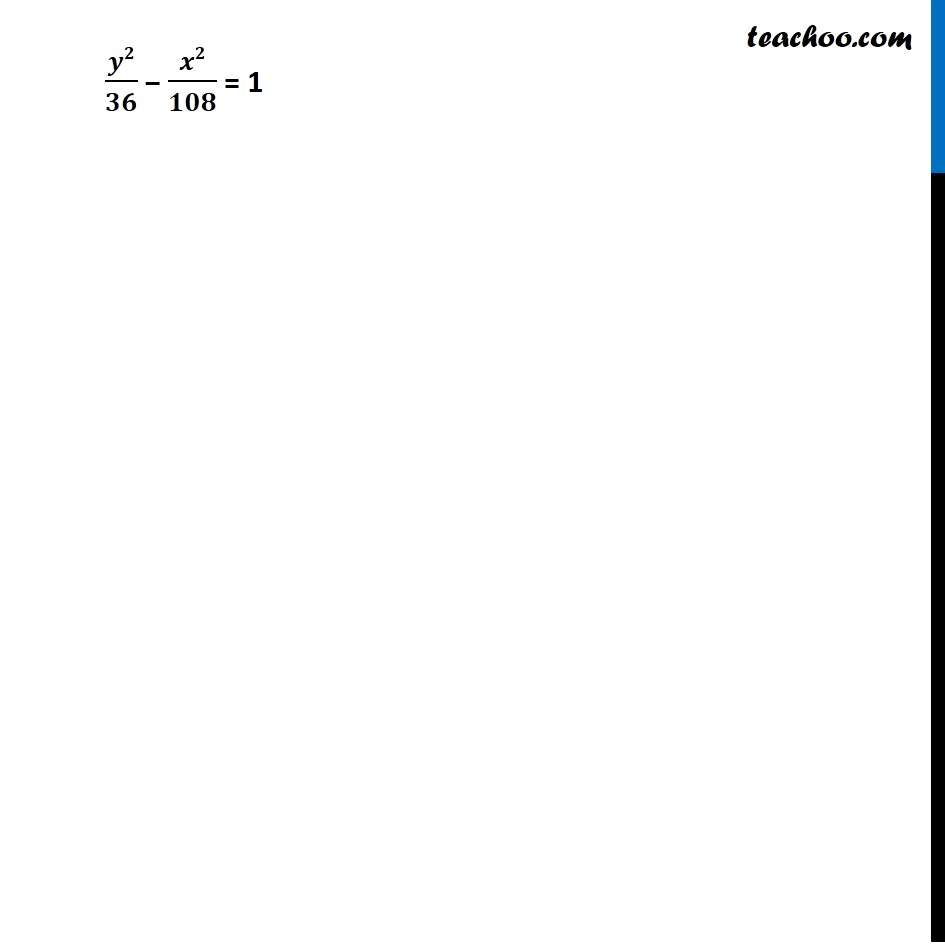Learn in your speed, with individual attention - Teachoo Maths 1-on-1 Class

### Transcript

Example 16 Find the equation of the hyperbola where foci are (0, ±12) and the length of the latus rectum is 36. We need to find equation of hyperbola Given foci (0, ±12) & length of latus rectum 36. Since foci is on the y−axis So required equation of hyperbola is 𝒚𝟐/𝒂𝟐 – 𝒙𝟐/𝒃𝟐 = 1 Now, Co-ordinates of foci = (0, ± c) & given foci = (0, ±12) So, (0, ± c) = (0, ±12) c = 12 We know that Length of latus rectum = 2𝑏2/𝑎 Given latus rectum = 36 36 = 2𝑏2/𝑎 36a = 2b2 2b2 = 36 a b2 = 36/2 𝑎 b2 = 18a We know that c2 = b2 + a2 Putting value of c & b2 (12)2 = 18a + a2 144 = 18a + a2 a2 + 18a = 144 a + 18a − 144 = 0 a2 + 24a − 6a − 144 = 0 a (a + 24) − 6 (a + 24) = 0 (a − 6) (a + 24) = 0 So , a = 6 or a = –24 Since ‘a’ is distance, it cannot be negative , So a = −24 is not possible ∴ a = 6, From (1) b2 = 18a Putting a = 6 b2 = 18 (6) b2 = 108 Required Equation of hyperbola is 𝑦2/𝑎2 − 𝑥2/𝑏2 = 1 Putting values 𝑦2/62 − 𝑥2/108 = 1 𝒚𝟐/𝟑𝟔 − 𝒙𝟐/𝟏𝟎𝟖 = 1